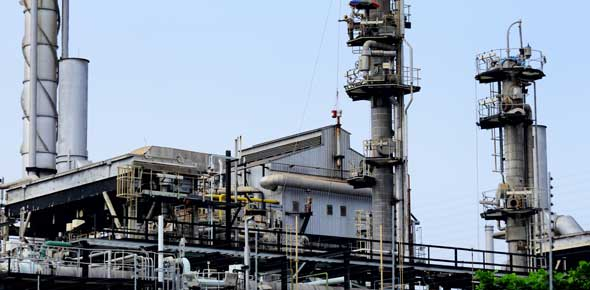10 Questions | Total Attempts: 434Settings• 1.
Which of the following statements is most accurate about inventory management?
• A.

Inventories and production must be managed together

• B.

Inventory is not important at the production planning level

• C.

Inventories are usually insignificant on the balance sheet

• D.

Inventory does not cost much to carry

• 2.
What is the name of materials used in the production process that do not become part of the product?
• A.

Raw materials

• B.

Work in process

• C.

Finished goods

• D.

Maintenance, repair, and operating supplies

• 3.
What is the name given to inventories of items that are purchased or manufactured in quantities greater than needed immediately?
• A.

Fluctuation inventory

• B.

Lot size inventory

• C.

Transportation inventory

• D.

Scheduled receipts

• 4.
Which of the following comapny objectives are in conflict?
• A.

Maximum customer service and low-cost plant operation

• B.

Low-cost plant operation and cash flow

• C.

Maximum inventory investment and customer service

• D.

Cash flow and profitability

• 5.
Which of the following costs will increase if order quantity is increased?
• A.

Annual cost of carrying inventory

• B.

Cost of ordering

• C.

Cost of manufacturing operations

• D.

Cost of customer service

• 6.
Which of the following are costs of carrying inventory?
• A.

Capital costs and production control costs

• B.

Capital costs and storage costs

• C.

Production control costs and purchase costs

• D.

• 7.
Which of the following are considered order costs?
• A.

Production control costs

• B.

Capital costs

• C.

Risk costs

• D.

Obsolescence costs

• 8.
Which of the folloiwng equations is correct?
• A.

Assets = Liabilities - Owners' Equity

• B.

Income = Revenue - Liabilities

• C.

Owners' Equity = Assets - Liabilities

• D.

Revenue = Cost of Goods Sold - General and Administrative Expenses

• 9.
If the annual cost of goods sold is \$10 million and the average inventory is \$2.5 million, what is the turns ratio?
• A.

\$7.5 million

• B.

.25

• C.

2.5

• D.

4

• 10.
Which of the following costs is relevant to inventory management decisions?
• A.

Run costs

• B.

Storage costs

• C.

Marketing costs

• D.

New product development costs

Related TopicsBack to top